# User guide for the noisySBM package

#### 20/10/2020

noisySBM is the accompanying R package of the article Powerful graph inference with false discovery rate control by Rebafka, Roquain and Villers available at https://arxiv.org/abs/1907.10176. It provides functions for generating data in the so-called noisy stochastic block model (NSBM), an estimation algorithm to fit parameters and cluster the nodes, as well as a powerful graph inference procedure.

The context is the following. We observe a dense graph where most interactions are due to noise. In practice the graph may be a similarity matrix, a correlation matrix or a matrix containing the values of a test statistic applied on all pairs of nodes. The aim is to infer the meaningful edges in the graph. This is obtained by a new random graph model and a new powerful graph inference procedure that comes with a control the number of flase discoveries.

The current version of the R package supports the entire analysis for undirected graphs in the Gaussian NSBM and the Gamma NSBM. The Poisson case as well as directed graphs are left for future developments.

library(noisySBM)

# 1 Noisy stochastic block model

We introduce a new random graph model suited for the problem of graph inference. In this model the observed matrix is a perturbation of an unobserved binary graph, which is the true graph to be inferred. The binary graph is chosen to be a stochastic block model (SBM) for its capacity to model graph heterogeneity. We refer to the new model as the noisy stochastic block model (NSBM).

The definition is stated for an undirected graph without self-loops, but extensions are straightforward. Let $$n\geq 2$$ be the number of nodes and $$\mathcal{A}=\{(i,j): 1\leq i <j\leq n\}$$ the set of all possible pairs of nodes. Denote $$Q$$ the number of latent node blocks. In the NSBM we observe edges $$X_{i,j}, (i,j)\in\mathcal{A}$$, and denote $$X=(X_{i,j})_{1\leq i,j\leq n}\in\mathbb R^{n^2}$$ the corresponding symmetric, real-valued observation matrix. The observations $$X_{i,j}, (i,j)\in\mathcal{A}$$, are generated by the following random layers:

1. First, a vector $$Z=(Z_1,\dots,Z_n)$$ of block memberships of the nodes is generated, such that $$Z_i$$, $$1\leq i\leq n$$, are independent with common distribution given by the probabilities $$\pi_q=\mathbb P(Z_1=q), q\in\{1,\dots,Q\},$$ for some parameter $$\pi=(\pi_q)_{q\in\{1,\dots,Q\}}\in (0,1)^Q$$ such that $$\sum_{q=1}^Q \pi_q=1$$.

2. Conditionally on $$Z$$, the variables $$A_{i,j}$$, $$(i,j)\in\mathcal A$$, are independent Bernoulli variables with parameter $$w_{Z_i,Z_j}$$, for some $$w=(w_{q,l})_{q,l\in\{1,\dots,Q\}}\in (0,1)^{Q^2}$$. As the graph is undirected, $$w$$ is symmetric. We denote $$A=(A_{i,j})_{1\leq i,j\leq n}$$ the corresponding symmetric adjacency matrix, which is a standard binary SBM.

3. Conditionally on $$(Z,A)$$, the observations $$X_{i,j}$$, $$(i,j)\in\mathcal A$$ are independent and $$X_{i,j}$$ is sampled from the distribution
$X_{i,j} \sim (1-A_{i,j}) g_{0,\nu_0} + A_{i,j} g_{\nu_{Z_i,Z_j}},$ where $$\nu_0\in \mathcal{V}_0$$ and $$\nu_{q,l}\in \mathcal{V}$$, $$1\leq q,l\leq Q$$ are unknown parameters, with $$\nu_{l,q}=\nu_{q,l}$$ for all $$q, l$$, and $$\{g_{0,\nu},\nu\in \mathcal{V}_0\}$$ and $$\{g_{\nu},\nu \in \mathcal{V}\}$$ are given parametric density families with non-empty open subsets $$\mathcal{V}_0\subset\mathbb R^{d_0}$$ and $$\mathcal{V}\subset\mathbb R^{d_1}$$.

The relation between $$A$$ and the observation $$X$$ is that missing edges ($$A_{i,j}=0$$) are replaced with pure random noise modeled by the density $$g_{0,\nu_0}$$, also called the null density, whereas in place of present edges ($$A_{i,j}=1$$) there is a signal or effect. The intensity of the signal depends on the block membership of the interacting nodes in the underlying SBM, modeled by the density $$g_{\nu_{q,l}}$$, also called alternative density for parameter $$\nu_{q,l}$$.

The unknown global model parameter is $$\theta=(\pi,w,\nu_0,\nu)$$, where $$\pi$$ and $$w$$ come from the SBM, $$\nu_0$$ denotes the null parameter and $$\nu=(\nu_{q,l})_{1\leq q, l\leq Q}\in \mathcal{V}^{Q^2}$$ denotes the parameters of the effects. Due to the symmetry of $$w$$ and $$\nu$$, we sometimes consider, with some abuse of notation, that the $$w$$ and $$\nu$$ belong to $$\mathbb R^{Q(Q+1)/2}$$ rather than $$\mathbb R^{Q^2}$$ .

Our main model is the Gaussian case. It is particularly suitable for modeling situations where the observations $$X_{i,j}$$ correspond to test statistics or correlations, which are known to be approximately Gaussian. The Gaussian NSBM corresponds to the NSBM with the following choice of the parametric density families: $\begin{equation}\label{gaussmodel} \{g_{0,\nu_0},\nu_0\in \mathcal{V}_0\}\subset\{\mathcal N(\mu,\sigma^2),\mu\in\mathbb R,\sigma>0\},\qquad\{g_{\nu},\nu \in \mathcal{V}\}\subset\{\mathcal N(\mu,\sigma^2),\mu\in\mathbb R,\sigma>0\}, \end{equation}$ where different choices for $$\mathcal{V}_0$$ and $$\mathcal{V}$$ lead to different variants. For example, often we assume that the mean of the null distribution is zero ($$\mu_0=0$$).

We may also consider the Gamma NSBM, where the parametric density families are chosen as follows: $\begin{equation}\label{gammamodel} \{g_{0,\nu},\nu\in \mathcal{V}_0\}=\{\text{Exp}(\nu_0),\nu_0>0\}, \:\:\:\{g_{\nu},\nu \in \mathcal{V}\}\subset\{\Gamma(a,b),a>0,b>0\}. \end{equation}$

In the Poisson NSBM both parametric families are the family of Poisson distirbutions: $\begin{equation}\label{possoinmodel} \{g_{0,\nu_0},\nu_0\in \mathcal{V}\}= \{g_{\nu},\nu \in \mathcal{V}\}=\{\text{Poi}(\nu),\nu>0\}. \end{equation}$

# 2 Model parameters and data generation of the NSBM with R

In the noisySBM package there is an overall parameters: the graph type (directed or undirected). Let us consider the case:

directed <- FALSE

The global model parameter $$\theta=(\pi,w,\nu_0,\nu)$$ of the NSBM is a list and its elements are pi, w, nu0 and nu.

theta <- list(pi=NULL, w=NULL, nu0=NULL, nu=NULL)

## 2.1 SBM parameters

Denote Q the number of latent blocks in the SBM. Say

 Q <- 2

The parameters pi and w are the parameters of the latent binary SBM, where pi is a Q-vector indicating the mean proportions of nodes per block. The vector pi has to be normalized:

theta$pi <- c(2,1)/3 Parameter w is a vector. For undirected graphs w is of length $$Q(Q+1)/2$$ with the following indexing $$w=(w_{1,1},w_{1,2},\dots,w_{1,Q},w_{2,2},\dots,w_{2,Q},w_{3,3},\dots,w_{Q,Q})$$. The parameter $$w_{q,l}\in(0,1)$$ is a Bernoulli parameter indicating the probability that a node in group $$q$$ is connected to a node in group $$l$$. For directed graphs w is of length $$Q^2$$ with the following indexing $$w=(w_{1,1},w_{1,2},\dots,w_{1,Q},w_{1,2},\dots,w_{2,Q},w_{1,3},\dots,w_{Q,Q})$$. In the directed case $$w_{q,l}\in(0,1)$$ is the probability of a directed edge from a node in group $$q$$ to a node in group $$l$$. For our undirected graph with two latent blocks, let us consider theta$w <- c(.8,.1,.9)

This means that both blocks are communities (as $$w_{1,1}=0.8$$ and $$w_{2,2}=0.9$$), as nodes have a large probability to be connected to nodes in the same block, and a low probability to connect to nodes in the other group ($$w_{1,2}=0.1$$).

## 2.2 Gaussian model

For the noisy layer, we have to choose a model, Gaussian, Gamma or Poisson.

In the Gaussian model, the null parameter nu0 is a vector of length 2, giving the mean and the standard deviation of the the Gaussian null distrubtion ($$\nu_0=(\nu_{0}^\text{mean},\nu_{0}^\text{sd})$$). Mostly, we choose the standard normal distribution:

theta$nu0 <- c(0,1) Parameter nu is a matrix with two columns: the first indicates Gaussian means $$\nu_{q,l}^\text{mean}$$, the second standard deviations $$\nu_{q,l}^\text{sd}$$ of the alternative distributions for the block pairs $$(q,l)$$. The number of rows of nu is identical to the length of w, that is, in the undirected case nu has $$Q(Q+1)/2$$ rows, otherwise $$Q^2$$. The rows are indexed like w, that is, $\nu^{\text{undirected}}=\begin{pmatrix}\nu_{1,1}\\\vdots\\\nu_{1,Q}\\\nu_{2,2} \\\vdots\\ \nu_{Q,Q} \end{pmatrix}\in\mathbb R^{Q(Q+1)/2\times 2}\qquad \nu^{\text{directed}}=\begin{pmatrix}\nu_{1,1}\\\nu_{1,Q}\vdots\\\\\nu_{2,1} \\\vdots\\ \nu_{Q,Q} \end{pmatrix}\in\mathbb R^{Q^2\times 2}\qquad \text{with } \nu_{q,l}=(\nu_{q,l}^\text{mean},\nu_{q,l}^\text{sd})\in\mathbb R^2.$ For our undirected model with two latent blocks, we choose theta$nu <- matrix(c(-1,5,-1, 1,1,1), ncol=2)
theta$nu ## [,1] [,2] ## [1,] -1 1 ## [2,] 5 1 ## [3,] -1 1 ### 2.2.1 Generate data To generate a data set with, say, $$n=10$$ nodes from the corresponding NSBM we use the function rnsbm(): obs <- rnsbm(n = 10, theta, modelFamily = 'Gauss') round(obs$dataMatrix, digits = 2)
##        [,1]  [,2]  [,3]  [,4]  [,5]  [,6]  [,7]  [,8]  [,9] [,10]
##  [1,]  0.00  1.50 -0.36  0.02  0.34 -1.46  1.20  0.46 -1.99 -0.63
##  [2,]  1.50  0.00 -0.99 -1.93  0.09 -0.22 -0.75  1.03 -0.35 -2.92
##  [3,] -0.36 -0.99  0.00 -1.14  0.61 -2.12 -0.67 -1.40  4.88 -1.52
##  [4,]  0.02 -1.93 -1.14  0.00 -0.40 -1.05  0.06  0.22 -0.70  0.32
##  [5,]  0.34  0.09  0.61 -0.40  0.00 -1.17 -1.63  1.85  0.19 -0.67
##  [6,] -1.46 -0.22 -2.12 -1.05 -1.17  0.00 -0.16 -1.65 -1.30 -0.18
##  [7,]  1.20 -0.75 -0.67  0.06 -1.63 -0.16  0.00  0.20  0.82 -0.80
##  [8,]  0.46  1.03 -1.40  0.22  1.85 -1.65  0.20  0.00 -2.41 -0.56
##  [9,] -1.99 -0.35  4.88 -0.70  0.19 -1.30  0.82 -2.41  0.00  0.81
## [10,] -0.63 -2.92 -1.52  0.32 -0.67 -0.18 -0.80 -0.56  0.81  0.00

We see that the generated matrix is symmetric, as we are in the undirected case. The function rnsbm() also provides the latent binary graph, named latentAdj, which is also symmetric:

obs$latentAdj ## [,1] [,2] [,3] [,4] [,5] [,6] [,7] [,8] [,9] [,10] ## [1,] 0 0 0 0 0 0 0 1 1 0 ## [2,] 0 0 1 1 1 1 1 0 0 1 ## [3,] 0 1 0 0 1 1 1 0 1 1 ## [4,] 0 1 0 0 0 1 1 0 0 1 ## [5,] 0 1 1 0 0 1 1 0 0 1 ## [6,] 0 1 1 1 1 0 1 0 0 1 ## [7,] 0 1 1 1 1 1 0 0 0 1 ## [8,] 1 0 0 0 0 0 0 0 1 0 ## [9,] 1 0 1 0 0 0 0 1 0 0 ## [10,] 0 1 1 1 1 1 1 0 0 0 as well as the latent blocks, names latentZ: obs$latentZ
##   2 1 1 1 1 1 1 2 2 1

A more convenient visualisation of the data is given by the function plotGraphs():

plotGraphs(obs$dataMatrix, binaryTruth=obs$latentAdj)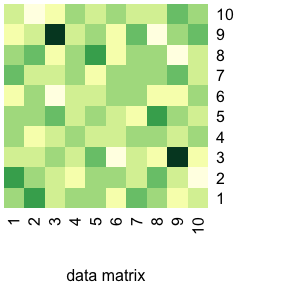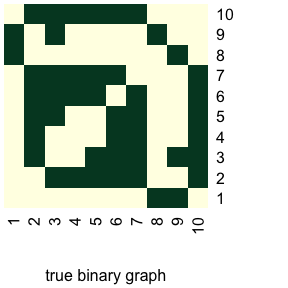## NULL

### 2.2.2 Directed graphs

To generate data from a directed graph, the parameters w and nu have to be adapted to length $$Q^2$$, say

theta$w <- c(.8,.1,.2,.9) theta$nu <- matrix(c(-1,5,10,-1, 1,1,1,1), ncol=2)
theta$nu ## [,1] [,2] ## [1,] -1 1 ## [2,] 5 1 ## [3,] 10 1 ## [4,] -1 1 To generate data from the directed NSBM, we set directed = TRUE: obs <- rnsbm(n = 10, theta, modelFamily = 'Gauss', directed = TRUE) plotGraphs(obs$dataMatrix, binaryTruth=obs$latentAdj)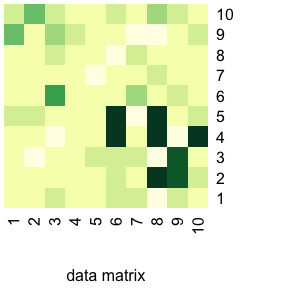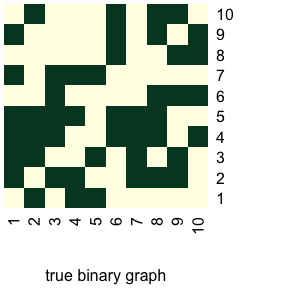## NULL round(obs$dataMatrix, digits = 2)
##        [,1]  [,2]  [,3]  [,4]  [,5]  [,6]  [,7]  [,8]  [,9] [,10]
##  [1,]  0.00 -1.35 -1.48 -0.96  0.72 -0.86 -1.27 -0.70  4.10  0.10
##  [2,] -0.88  0.00 -1.64 -0.33  1.31 -0.79 -0.46 -0.79 -0.59  3.79
##  [3,]  0.08 -1.53  0.00 -3.04 -1.07  4.80 -0.56  0.13  1.85  0.75
##  [4,] -0.85 -0.75 -0.52  0.00 -1.11 -1.34 -0.39 -0.88  0.34 -0.18
##  [5,] -1.47 -0.46  0.06 -1.15  0.00 -0.56 -1.97 -0.91 -1.39 -1.14
##  [6,]  0.63  0.87  1.50 10.31 10.91  0.00 -0.29 -2.02 -0.30  0.70
##  [7,]  0.17 -1.21  1.06 -1.37 -3.11  1.65  0.00  1.02 -2.52 -0.48
##  [8,] -2.40 10.66 -1.86 10.70  9.78 -1.29  0.56  0.00 -2.11  1.88
##  [9,]  0.87  9.12  8.65 -1.61 -0.07  0.09 -1.00 -0.42  0.00  0.10
## [10,] -1.11  0.90 -0.26  9.57  1.17 -0.76 -0.72 -0.44  0.51  0.00
obs$latentAdj ## [,1] [,2] [,3] [,4] [,5] [,6] [,7] [,8] [,9] [,10] ## [1,] 0 1 1 1 1 0 1 0 1 0 ## [2,] 1 0 1 1 1 0 0 0 0 1 ## [3,] 0 1 0 1 1 1 1 0 0 0 ## [4,] 1 1 0 0 1 0 1 0 0 0 ## [5,] 1 0 1 0 0 0 1 0 0 0 ## [6,] 0 0 0 1 1 0 0 1 1 1 ## [7,] 0 1 1 1 1 0 0 0 0 0 ## [8,] 0 1 0 1 1 1 0 0 1 1 ## [9,] 0 1 1 0 0 1 0 1 0 1 ## [10,] 0 0 0 1 0 1 0 1 1 0 Now the matrices are no longer symmetric. ## 2.3 Gamma model As the Gamma distribution also has two parameters, nu0 is a vector of length 2 and nu is a 2-column matrix as in the Gaussian case. Let us take as an example an undirectd graph in a model with $$Q=3$$ latent blocks with equiprobable blocks: theta$pi <- rep(1/3, 3)

Suppose that inter-block probabilities are high, while intra-block probabilities are low:

theta$w <- c(.1, .8, .8, .1, .8, .1) The null distribution is the exponential distribution Exp$$(2)$$: theta$nu0 <- c(1,2)

For the alternative distributions we also choose exponentials:

theta$nu <- matrix(c(1, 1, 1, 1, 1, 1, 1, .5, .5, 1, .5, 1), ncol=2) theta$nu
##      [,1] [,2]
## [1,]    1  1.0
## [2,]    1  0.5
## [3,]    1  0.5
## [4,]    1  1.0
## [5,]    1  0.5
## [6,]    1  1.0

We generate a graph with 10 nodes:

obs <- rnsbm(n = 10, theta, modelFamily = 'Gamma')
plotGraphs(obs$dataMatrix, binaryTruth=obs$latentAdj)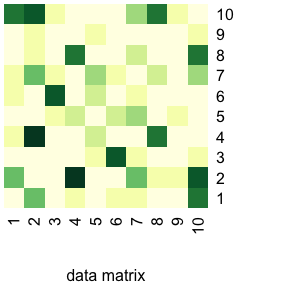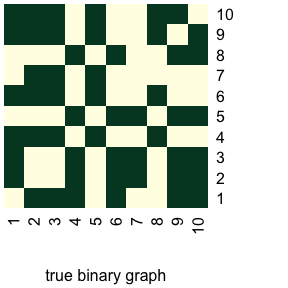## NULL
round(obs$dataMatrix, digits = 2) ## [,1] [,2] [,3] [,4] [,5] [,6] [,7] [,8] [,9] [,10] ## [1,] 0.00 2.73 0.05 0.83 0.11 1.27 0.87 0.17 0.46 4.55 ## [2,] 2.73 0.00 0.07 5.92 0.40 0.08 2.76 0.86 0.81 4.71 ## [3,] 0.05 0.07 0.00 0.24 1.21 5.09 1.22 0.24 0.41 1.12 ## [4,] 0.83 5.92 0.24 0.00 1.96 0.04 0.41 4.59 0.02 0.47 ## [5,] 0.11 0.40 1.21 1.96 0.00 1.36 2.22 0.33 1.17 0.53 ## [6,] 1.27 0.08 5.09 0.04 1.36 0.00 0.94 0.62 0.16 0.46 ## [7,] 0.87 2.76 1.22 0.41 2.22 0.94 0.00 1.76 0.05 2.07 ## [8,] 0.17 0.86 0.24 4.59 0.33 0.62 1.76 0.00 0.53 4.07 ## [9,] 0.46 0.81 0.41 0.02 1.17 0.16 0.05 0.53 0.00 0.67 ## [10,] 4.55 4.71 1.12 0.47 0.53 0.46 2.07 4.07 0.67 0.00 obs$latentAdj
##       [,1] [,2] [,3] [,4] [,5] [,6] [,7] [,8] [,9] [,10]
##  [1,]    0    1    1    1    0    1    0    0    1     1
##  [2,]    1    0    0    1    0    1    1    0    1     1
##  [3,]    1    0    0    1    0    1    1    0    1     1
##  [4,]    1    1    1    0    1    0    0    1    0     0
##  [5,]    0    0    0    1    0    1    1    0    1     1
##  [6,]    1    1    1    0    1    0    0    1    0     0
##  [7,]    0    1    1    0    1    0    0    0    0     0
##  [8,]    0    0    0    1    0    1    0    0    1     1
##  [9,]    1    1    1    0    1    0    0    1    0     1
## [10,]    1    1    1    0    1    0    0    1    1     0
obs$latentZ ##  3 2 2 1 2 1 1 2 1 1 ## 2.4 Poisson model The Poisson distribution has a single parameter, so that nu0 is a scalar and nu becomes a one-column matrix. As an example consider a directed $$Q=1$$-block Poisson NSBM with parameters: theta <- list(pi=1, w=.5, nu0=1, nu=5) We generate a graph with 10 nodes: obs <- rnsbm(n = 10, theta, modelFamily = 'Poisson', directed = TRUE) plotGraphs(obs$dataMatrix, binaryTruth=obs$latentAdj)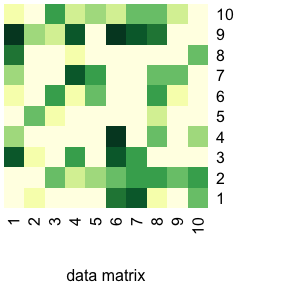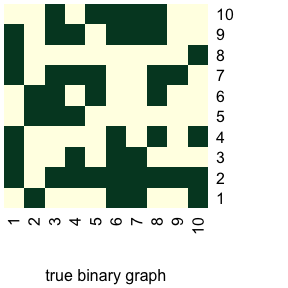## NULL round(obs$dataMatrix)
##       [,1] [,2] [,3] [,4] [,5] [,6] [,7] [,8] [,9] [,10]
##  [1,]    0    1    8    4    0    2    4    7    9     2
##  [2,]    2    0    2    0    5    1    0    0    4     1
##  [3,]    0    5    0    1    2    6    1    0    3     6
##  [4,]    1    3    6    0    1    2    8    2    8     3
##  [5,]    0    4    0    1    0    5    6    1    1     4
##  [6,]    7    5    8    9    1    0    0    0    9     3
##  [7,]    8    6    6    0    1    1    0    1    8     5
##  [8,]    2    6    0    5    3    6    5    0    7     5
##  [9,]    0    5    0    0    1    2    5    1    0     3
## [10,]    5    6    0    4    0    1    1    5    0     0
obs$latentAdj ## [,1] [,2] [,3] [,4] [,5] [,6] [,7] [,8] [,9] [,10] ## [1,] 0 1 1 1 0 0 1 1 1 0 ## [2,] 1 0 0 0 1 1 0 0 0 0 ## [3,] 0 1 0 0 1 1 1 0 1 1 ## [4,] 0 1 1 0 1 0 1 0 1 0 ## [5,] 0 1 0 0 0 1 1 0 0 1 ## [6,] 1 1 1 1 0 0 0 0 1 1 ## [7,] 1 1 1 0 0 0 0 0 1 1 ## [8,] 0 1 0 1 0 1 1 0 1 1 ## [9,] 0 1 0 0 0 0 1 0 0 0 ## [10,] 1 1 0 1 0 0 0 1 0 0 obs$latentZ
##   1 1 1 1 1 1 1 1 1 1

# 3 Estimation algorithm

## 3.1 Basic function call fitNSBM() and output

The function fitNSBM() fits the parameters of a NSBM to a data set in different models. The function applies to a symmetric real-valued square matrix dataMatrix. Let us see a basic example:

set.seed(1)
theta1 <- list(pi=c(.5,.5), w=c(.8,.1,.2), nu0=c(0,1), nu=matrix(c(-1,5,10, 1,1,1), ncol=2))
obs1 <- rnsbm(n=30, theta1)
res_gauss <- fitNSBM(obs1$dataMatrix, nbCores=2) fitNSBM() applies the VEM-algorithm to estimate model parameters and compute a node clustering. By default, the algorithm explores different SBM models starting from a model with a single latent block (Q=1) up to 4 blocks or more if appropriate. The output of fitNSBM() is a list with estimation results for all visited models (for Qmin=1 to Qmax=4 (or more)). Here, for Q=2 for instance, we obtain the estimates of the model parameters $$\theta$$ by res_gauss[]$theta
## $w ##  0.8336686 0.1025082 0.1392889 ## ##$nu0
##  0.00000 1.02319
##
## $nu ## [,1] [,2] ## [1,] -1.172879 0.8221477 ## [2,] 5.089987 0.9116246 ## [3,] 10.233534 0.9057642 ## ##$pi
##  0.4327493 0.5672507

A node clustering is given by

res_gauss[]$clustering ##  2 2 1 1 2 1 1 1 1 2 2 2 1 2 1 2 1 1 2 2 1 2 1 2 2 2 2 2 1 2 The list element sbmParam contains further results concerning the latent binary SBM, namely the number of latent blocks: res_gauss[]$sbmParam$Q ##  2 the variational parameters, i.e. the probabilities that a node belongs to one of the latent blocks: res_gauss[]$sbmParam$clusterProba ## [,1] [,2] [,3] [,4] [,5] [,6] [,7] ## [1,] 0.0000001 0.0000001 0.9999999 0.9999999 0.0000001 0.9999999 0.9999999 ## [2,] 0.9999999 0.9999999 0.0000001 0.0000001 0.9999999 0.0000001 0.0000001 ## [,8] [,9] [,10] [,11] [,12] [,13] [,14] ## [1,] 0.9999999 0.9999999 0.0000001 0.0000001 0.0000001 0.9999999 0.0000001 ## [2,] 0.0000001 0.0000001 0.9999999 0.9999999 0.9999999 0.0000001 0.9999999 ## [,15] [,16] [,17] [,18] [,19] [,20] [,21] ## [1,] 0.9999999 0.0000001 0.9998480401 0.9999999 0.0000001 0.1307727 0.8518565 ## [2,] 0.0000001 0.9999999 0.0001519599 0.0000001 0.9999999 0.8692273 0.1481435 ## [,22] [,23] [,24] [,25] [,26] [,27] [,28] ## [1,] 0.0000001 0.9999999 0.0000001 0.0000001 0.0000001 0.0000001 0.0000001 ## [2,] 0.9999999 0.0000001 0.9999999 0.9999999 0.9999999 0.9999999 0.9999999 ## [,29] [,30] ## [1,] 0.9999999 0.0000001 ## [2,] 0.0000001 0.9999999 and the conditional probabilities of an edge between a pair of nodes given the block memberships of the nodes: res_gauss[]$sbmParam$edgeProba[,1:5] ## [,1] [,2] [,3] [,4] [,5] ## [1,] 7.692067e-02 9.058548e-01 5.283000e-02 7.476060e-01 6.906621e-01 ## [2,] 2.045408e-04 4.729178e-11 4.974843e-04 8.095808e-09 2.256252e-08 ## [3,] 2.867288e-16 1.460504e-16 3.646087e-16 9.331119e-17 9.216202e-17 These probabilities correspond to $$1-l\text{-values}$$, which are used in the test procedure for graph inference. The dimension of sbmParam$edgeProba is $$Q(Q+1)/2$$ times $$n(n-1)/2$$ (in the undirected case):

dim(res_gauss[]$sbmParam$edgeProba)
##    3 435

that is, the rows correspond to group pairs $$(q,l)$$ and the columns to node pairs $$(i,j)$$.

Moreover, sbmParam contains the values of the integrated classification criterion:

res_gauss[]$sbmParam$ICL
##  -1647.502

Finally, the list element convergence provides details on the convergence of the algorithm:

res_gauss[]$convergence ##$J
##  -762.9652
##
## $complLogLik ##  -791.6735 ## ##$converged
##  TRUE
##
## $nbIter ##  82 ## 3.2 Models for edge distributions For the edges different distributions can be considered. The noisySBM package supports the Gaussian NSBM and the Gamma NSBM. In the Gaussian case a number of different restrictions on the parameter space are implemented. ### 3.2.1 Gaussian NSBM By default, fitNSBM() considers a Gaussian NSBM with • model='Gauss0', where the null distribution is a centered normal distribution with $$\nu_{0}^{\text{mean}}=0$$ and standard deviation $$\nu_{0}^{\text{sd}}\in\mathbb R_+$$ to be estimated, and all parameters $$\nu_{q,l}=(\nu_{q,l}^\text{mean},\nu_{q,l}^\text{sd})$$ for $$1\leq q\leq l\leq Q$$ of the alternative Gaussian distributions are estimated without any constraint. Other choices for model in the Gaussian case are the following: • Gauss: all Gaussian parameters of the null and the alternative distributions are unknown ; this is the Gaussian model with the maximum number of unknown parameters ($$\nu_{0}^{\text{mean}}\in\mathbb R$$, $$\nu_{q,l}^{\text{mean}}\in\mathbb R$$, $$\nu_{0}^{\text{sd}}\in\mathbb R_+$$, $$\nu_{q,l}^{\text{sd}}\in\mathbb R_+$$ for all $$q\leq l$$). • Gauss01: compared to Gauss, the null distribution is set to $$\mathcal N(0,1)$$ ($$\nu_0^{\text{mean}}=0$$, $$\nu_0^{\text{sd}}=1$$)) • GaussEqVar: compared to Gauss, all Gaussian variances (of both the null and the alternative) are supposed to be equal, but unknown ($$\nu_0^{\text{sd}}=\nu_{q,l}^{\text{sd}}\in\mathbb R_+$$ for all $$q\leq l$$). • Gauss0EqVar: compared to GaussEqVar, the mean of the null distribution is set to 0 ($$\nu_0^{\text{mean}}=0$$). • Gauss0Var1: compared to Gauss, all Gaussian variances are set to 1 and the null distribution is set to $$\mathcal N(0,1)$$ ($$\nu_0^{\text{mean}}=0$$, $$\nu_0^{\text{sd}}=\nu_{q,l}^{\text{sd}}=1$$) • Gauss2distr: the alternative distribution is a single Gaussian distribution, i.e. the block memberships of the nodes do not influence on the alternative distribution (all $$\nu_{q,l}$$ are identical). • GaussAffil: compared to Gauss, for the alternative distribution, there’s a distribution for inter-group and another for intra-group interactions (there are parameters $$\nu_{\text{in}}$$ and $$\nu_{\text{out}}$$ such that $$\nu_{q,q}=\nu_{\text{in}}$$ for every $$q$$, and $$\nu_{q,l}=\nu_{\text{out}}$$ for every $$q< l$$). ### 3.2.2 Gamma NSBM The estimation procedure fitNSBM() supports two exponential or Gamma models: • Exp: the null and the alternatives are all exponential distributions with unknown parameters (i.e. they are Gamma distributions with shape parameter equal to one and unknown scale parameter) • ExpGamma: the null distribution is exponential with unknown parameter, the alterantive distribution are Gamma distributions where both parameters are unknown. Let us consider the following example: theta2 <- list(pi=rep(1/2, 2), w=c(.2, .8, .2), nu0=c(1,5), nu=matrix(c(1, 1, 1, 1, 1/3, 1), ncol=2)) set.seed(2) obs2 <- rnsbm(n=60, theta2, modelFamily='Gamma') Let us fit both Gamma models to the data : res_exp <- fitNSBM(obs2$dataMatrix, model='Exp', nbCores = 2)

set.seed(3)
res_gamma <- fitNSBM(obs2$dataMatrix, model='ExpGamma', nbCores = 2) The parameter estimates obtained for $$Q=2$$ are close to the true parameter values in both cases: Q <- 2 res_exp[[Q]]$theta
## $w ##  0.2572669 0.7285259 0.1674702 ## ##$nu0
##  1.00000 5.09704
##
## $nu ## [,1] [,2] ## [1,] 1 1.1857191 ## [2,] 1 0.3159445 ## [3,] 1 0.9510055 ## ##$pi
##  0.4666667 0.5333333
res_gamma[[Q]]$theta ##$w
##  0.1787426 0.6857469 0.1441840
##
## $nu0 ##  1.000000 4.879132 ## ##$nu
##          [,1]      [,2]
## [1,] 1.522966 1.4000245
## [2,] 1.205248 0.3603533
## [3,] 1.064406 0.9241991
##
## $pi ##  0.4666667 0.5333333 ## 3.3 Model selection When the algorithm explores several model sizes $$Q$$, then model selection can be performed via the ICL criterion. The function getBestQ() gives the best model size $$\hat Q$$ that maximizes the ICL: getBestQ(res_gauss) ##$Q
##  3
##
## $ICL ##  -1625.099 Typically, the ICL curve is a concave function, as we can see by applying function plotICL: plotICL(res_gauss)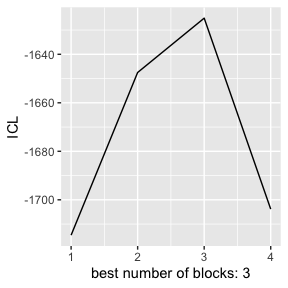Likewise, for our exponential data, we obtain: getBestQ(res_exp) ##$Q
##  2
##
## $ICL ##  -4034.005 getBestQ(res_gamma) ##$Q
##  2
##
## $ICL ##  -3855.48 and for the ICL curves: dfICLexp <- data.frame(ICL = sapply(res_exp, function(el) el$sbmParam$ICL), Q = sapply(res_exp, function(el) el$sbmParam$Q), model='Exp') dfICLgam <- data.frame(ICL = sapply(res_gamma, function(el) el$sbmParam$ICL), Q = sapply(res_gamma, function(el) el$sbmParam$Q), model='ExpGamma') dfICL <- rbind(dfICLexp, dfICLgam) ggplot(dfICL, aes(x=Q, y=ICL, group=model, colour=model) ) + geom_line() + xlim(1,4)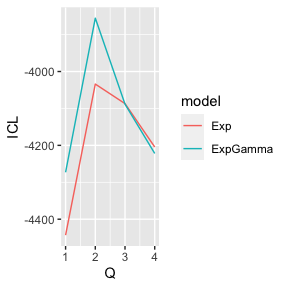The argument sbmSize of fitNSBM() is used to precise the models to be explored. When sbmSize$Qmin and sbmSize$Qmax are specified, then only this range of models is explored. For a single model size, do e.g. res4 <- fitNSBM(obs1$dataMatrix, sbmSize=list(Qmin=2, Qmax=2), nbCores=2)

## 3.4 Initialization

The argument initParam is a list and you may change default values to increase or descrease the number of initial points of the algorithm.

## 3.5 Save output

To directly save the output, a filename has to be precised in the call of fitNSBM().

## 3.6 Parlallel computing

By default, computations are parallelized using the maximum number of available cores. However, the argument nbCores can be used to customize this choice.

## 3.7 Node clustering

Node clusterings can be compared vie the adjusted Rand index. The package provides a function ARI() to evaluate this indicator. On simulated data we can compare to the true clustering:

ARI(res_gauss[]$clustering, obs1$latentZ)
##  0.8891908

To compare two node clusterings with different number of clusters:

ARI(res_gauss[]$clustering, res_gauss[]$clustering)
##  0.8663651

For the example for the Gamma NSBM, we find that the clusterings are perfect in both models (Exp and ExpGamma):

ARI(res_exp[]$clustering, obs2$latentZ)
##  1
ARI(res_gamma[]$clustering, obs2$latentZ)
##  1

# 4 Graph inference by multiple testing

Graph inference is done by the function graphInference(). The function provides the estimated binary graph at level alpha using the approach of q-values in a NSBM. The arguments are the observed dataMatrix, a clustering, a parameter estimate theta, a significance level alpha and the model modelFamily. It returns a list containing the inferred graph A:

resGraph <- graphInference(obs1$dataMatrix, res_gauss[]$clustering, res_gauss[]$theta, alpha = .05, modelFamily='Gauss') resGraph$A[1:5, 1:5]
##      [,1] [,2] [,3] [,4] [,5]
## [1,]    0    0    0    0    0
## [2,]    0    0    0    1    0
## [3,]    0    0    0    1    0
## [4,]    0    1    1    0    0
## [5,]    0    0    0    0    0

The ouptut also contains the q-values:

resGraph$qval[1:10] ##  0.6530847 0.6127403 0.1729543 0.6530847 0.4992396 0.5169365 0.2844466 ##  0.3909315 0.6530847 0.6530847 which is a vector of length $$n(n-1)/2$$: length(resGraph$qval)
##  435

To visualize the result, we can use again the function graphPlots() that also returns the FDR and TDR:

plotGraphs(obs1$dataMatrix, resGraph$A, obs1$latentAdj)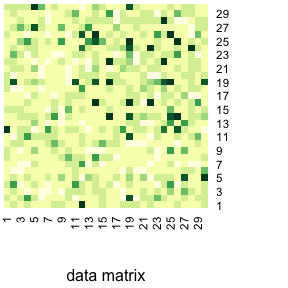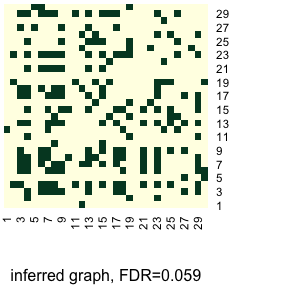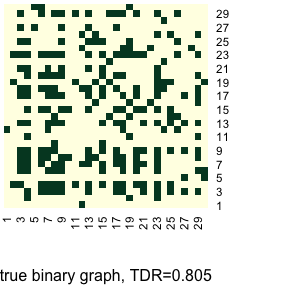##$FDR
##  0.05940594
##
## $TDR ##  0.8050847 The graph inference procedure is also implemented in the Gamma model, but only in case where the shape parameter of all Gamma distributions under the null and under the alternative is the same, so for instance in the model where all distributions are exponential distributions. resGraph2 <- graphInference(obs2$dataMatrix, res_exp[]$clustering, res_exp[]$theta, alpha = .05, modelFamily='Gamma')
resGraph2$A[1:5, 1:5] ## [,1] [,2] [,3] [,4] [,5] ## [1,] 0 1 0 0 1 ## [2,] 1 0 0 1 0 ## [3,] 0 0 0 1 0 ## [4,] 0 1 1 0 0 ## [5,] 1 0 0 0 0 The ouptut also contains the q-values: resGraph2$qval[1:10]
##   5.864995e-07 2.776895e-01 2.508740e-01 6.342863e-04 2.655163e-01
##   4.825911e-02 1.583784e-03 4.200489e-01 4.114394e-04 1.662359e-01

which is a vector of length $$n(n-1)/2$$:

length(resGraph2$qval) ##  1770 To visualize the result, we can use again the function graphPlots() that also returns the FDR and TDR: plotGraphs(obs2$dataMatrix, resGraph2$A, obs2$latentAdj)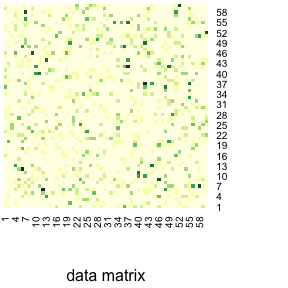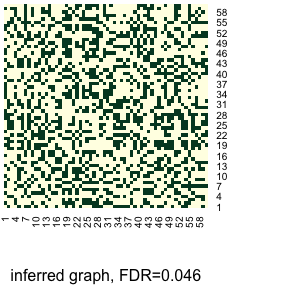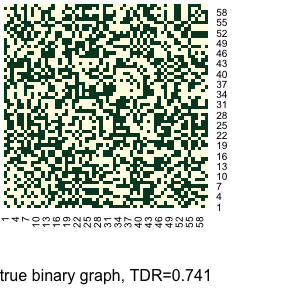## $FDR ##  0.04626866 ## ##$TDR
##  0.7412993